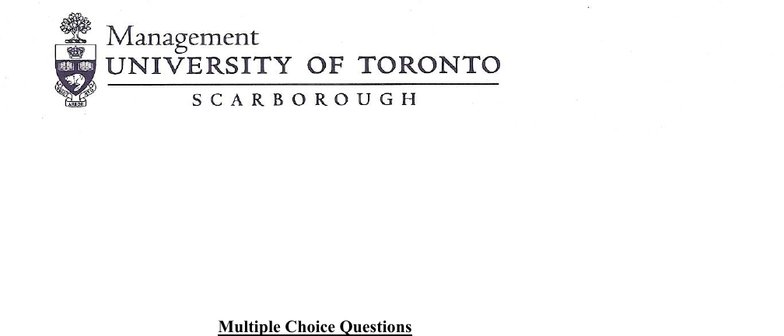# MGEB12H3 Lecture Notes - Lecture 1: Central Limit Theorem, Sampling Distribution, Statistical Inference

94 views12 pages
School
UTSC
Department
Economics for Management Studies
Course
MGEB12H3
ProfessorMGEB12 – QUANTITATIVE METHODS IN ECONOMICS-II
Problem Set – 0 (Sampling Distribution)
Note: Although sampling distribution is covered in MGEB11, it is the cornerstone of
statistical inference (what you learn in MGEB12). Make sure you go through this
problem set carefully.
Multiple Choice Questions
1. In a large population of adults, the mean IQ is 112 with a standard deviation of 20.
Suppose 200 adults are randomly selected for a market research campaign. What is the
probability that the sample mean IQ is greater than 110?
A. 0.579
B. 0.921
C. 0.421
D. 0.079
E. 0.1423
2. The marks in a statistics course are normally distributed with a population standard
deviation of 10 marks. If the probability that the sample mean of 25 randomly selected
students’ marks is greater than 75 is 0.0228, the population mean of the marks must be
closest to:
A. 67
B. 69
C. 70
D. 71
E. 73
3. Which of the following statements is consistent with the Central Limit Theorem?
A. When µ and σ are known, the population will be approximately normally distributed.
B. If a population has µ and σ, a sample from that population will be normally distributed if
the sample size is large enough.
C. When we know σ, the variation in the sample means will be equal to that of the
population.
D. Means of samples for n=30 from a uniform distribution will be approximately normally
distributed.
E. None of the above
Unlock document

This preview shows pages 1-3 of the document.
Unlock all 12 pages and 3 million more documents.

2
4. According to the Central Limit Theorem:
A. All variables have approximately bell-shaped sample distributions if a random sample
contains at least 30 observations
B. Population distributions are normal whenever the population size is large
C. For large random samples, the sampling distribution of the sample mean ( ) is
approximately normal, regardless of the shape of the population distribution.
D. The sampling distribution looks more like the population distribution as the sample size
increases
E. All of the above
5. The weights of bricks have a Normal distribution with mean 110.0 grams and standard
deviation 0.4 grams. If the weights of 22 randomly selected bricks are measured, the
probability that the resulting point estimate of will be in the interval (109.8, 110.2) is
approximately:
Note: The sample mean is a point estimate of the population mean.
A. 0.94
B. 0.95
C. 0.96
D. 0.97
E. 0.98
6. A group of students weigh on average 70 Kg, with a standard deviation of 5 Kg. If a
group of 50 students are sampled at random, the probability that their total weight does not
exceed 3600 Kg is:
A. 0.9961
B. 0.9977
C. 0.9989
D. 0.9952
E. 0.9931
7. A restaurant chain has 256 booths (tables). For Monday breakfast it has found that on
average only 116 of the booths are in use by customers. What is the probability (rounded to
the nearest hundredth) that on a given Monday less than 100 booths are used?
A. Less than 0.01
B. 0.02
C. 0.05
D. 0.39
E. None of the above
8. A recent study concluded that 36% of fish in a certain river were mature females. A
sample of 200 fish are taken randomly from the same river. What is the probability that the
sample proportion of mature female fish will be within +/-.05 of the actual population
proportion?
Unlock document

This preview shows pages 1-3 of the document.
Unlock all 12 pages and 3 million more documents.

3
A. 0.2345
B. 0.4371
C. 0.6451
D. 0.8592
E. 0.9621
9. In a recent poll a candidate enjoyed 50% of votes in Toronto. Suppose that a random
sample of 25 people from Toronto is selected. Use the normal approximation to the
binomial distribution and calculate the probability that between 10- 15 people of the
sampled people voted for this candidate:
A. 0.3217
B. 0.6844
C. 0.5123
D. 0.3688
E. 0.3312
10. The Heart Association claims that 90% of adults over 25 years of age cannot meet the
minimum requirements of the Physical Fitness. A random sample of 400 adults is selected.
The probability that at least 50 of these 400 adults meet the minimum requirements is:
A. 0.1583
B. 0.8417
C. 0.9429
D. 0.0475
E. None of the above
Unlock document

This preview shows pages 1-3 of the document.
Unlock all 12 pages and 3 million more documents.